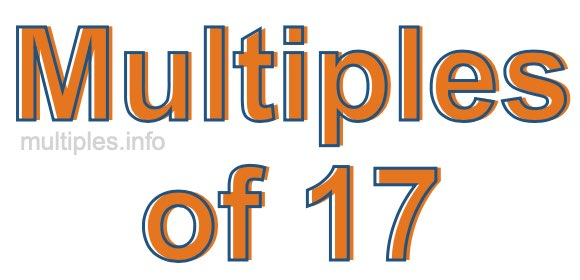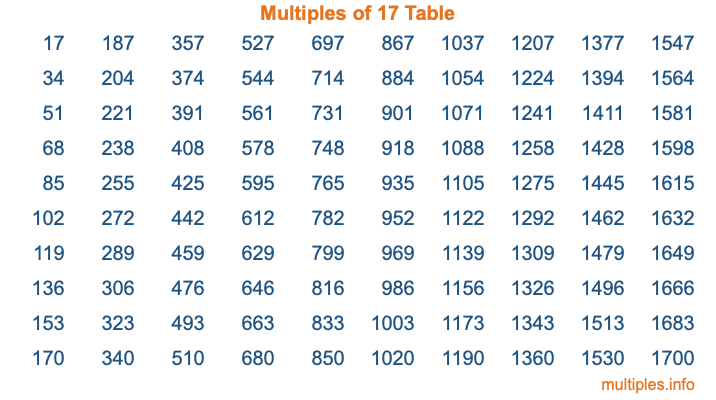Multiples of 17Welcome to the Multiples of 17 page. Here we will first teach you everything you will ever need to know about the multiples of 17, and then give you a study guide summary of everything we taught you to make sure you remember it all. Use this page to look up facts and learn information about the multiples of 17. This page will make you a multiples of seventeen expert!

Definition of Multiples of 17
Multiples of 17 are all the numbers that when divided by 17 equal an integer. Each of the multiples of 17 are called a multiple. A multiple of 17 is created by multiplying 17 by an integer.

Therefore, to create a list of multiples of 17, you start with 1 multiplied by 17, then 2 multiplied by 17, then 3 multiplied by 17, and so on for as long as you want. Thus, the list of the first five multiples of 17 is 17, 34, 51, 68, and 85. To see a larger list of multiples of 17, see the printable image of Multiples of 17 further down on this page. We also have a category where you can choose any nth multiple of 17.

Multiples of 17 Checker
The Multiples of 17 Checker below checks to see if any number of your choice is a multiple of 17. In other words, it checks to see if there is any number (integer) that when multiplied by 17 will equal your number. To do that, we divide your number by 17. If the the quotient is an integer, then your number is a multiple of 17.

Is  a multiple of 17?

Least Common Multiple of 17 and ...
A Least Common Multiple (LCM) is the lowest multiple that two or more numbers have in common. This is also called the smallest common multiple or lowest common multiple and is useful to know when you are adding our subtracting fractions. Enter one or more numbers below (17 is already entered) to find the LCM.

Check out our LCM Calculator if you need more details about the Least Common Multiple or if you need the LCM for different numbers for adding and subtraction fractions.

nth Multiple of 17
As we stated above, 17 is the first multiple of 17, 34 is the second multiple of 17, 51 is the third multiple of 17, and so on. Enter a number below to find the nth multiple of 17.

th multiple of 17

Multiples of 17 vs Factors of 17
17 is a multiple of 17 and a factor of 17, but that is where the similarities end. All postive multiples of 17 are 17 or greater than 17. All positive factors of 17 are 17 or less than 17.

Below is the beginning list of multiples of 17 and the factors of 17 so you can compare:

Multiples of 17: 17, 34, 51, 68, 85, etc.

Factors of 17: 1, 17

As you can see, the multiples of 17 are all the numbers that you can divide by 17 to get a whole number. The factors of 17, on the other hand, are all the whole numbers that you can multiply by another whole number to get 17.

It's also interesting to note that if a number (x) is a factor of 17, then 17 will also be a multiple of that number (x).

Multiples of 17 vs Divisors of 17
The divisors of 17 are all the integers that 17 can be divided by evenly. Below is a list of the divisors of 17.

Divisors of 17: 1, 17

The interesting thing to note here is that if you take any multiple of 17 and divide it by a divisor of 17, you will see that the quotient is an integer.

Multiples of 17 Table
Below is an image of the first 100 multiples of 17 in a table. The table is in chronological order, column by column. The first column has the first ten multiples of 17, the second column has the next ten multiples of 17, and so on.The Multiples of 17 Table is also referred to as the 17 Times Table or Times Table of 17. You are welcome to print out our table for your studies.

Negative Multiples of 17
Although not often discussed or needed in math, it is worth mentioning that you can make a list of negative multiples of 17 by multiplying 17 by -1, then by -2, then by -3, and so on, to get the following list of negative multiples of 17:

-17, -34, -51, -68, -85, etc.

Multiples of 17 Summary
Below is a summary of important Multiples of 17 facts that we have discussed on this page. To retain the knowledge on this page, we recommend that you read through the summary and explain to yourself or a study partner why they hold true.

There are an infinite number of multiples of 17.

A multiple of 17 divided by 17 will equal a whole number.

17 divided by a factor of 17 equals a divisor of 17.

The nth multiple of 17 is n times 17.

The largest factor of 17 is equal to the first positive multiple of 17.

17 is a multiple of every factor of 17.

17 is a multiple of 17.

A multiple of 17 divided by a divisor of 17 equals an integer.

17 divided by a divisor of 17 equals a factor of 17.

Any integer times 17 will equal a multiple of 17.

Multiples of a Number
Here you can get the multiples of another number, all with the same attention to detail as we did for multiples of 17 on this page.

Multiples of
Multiples of 18
Did you find our page about multiples of seventeen educational? Do you want more knowledge? Check out the multiples of the next number on our list!